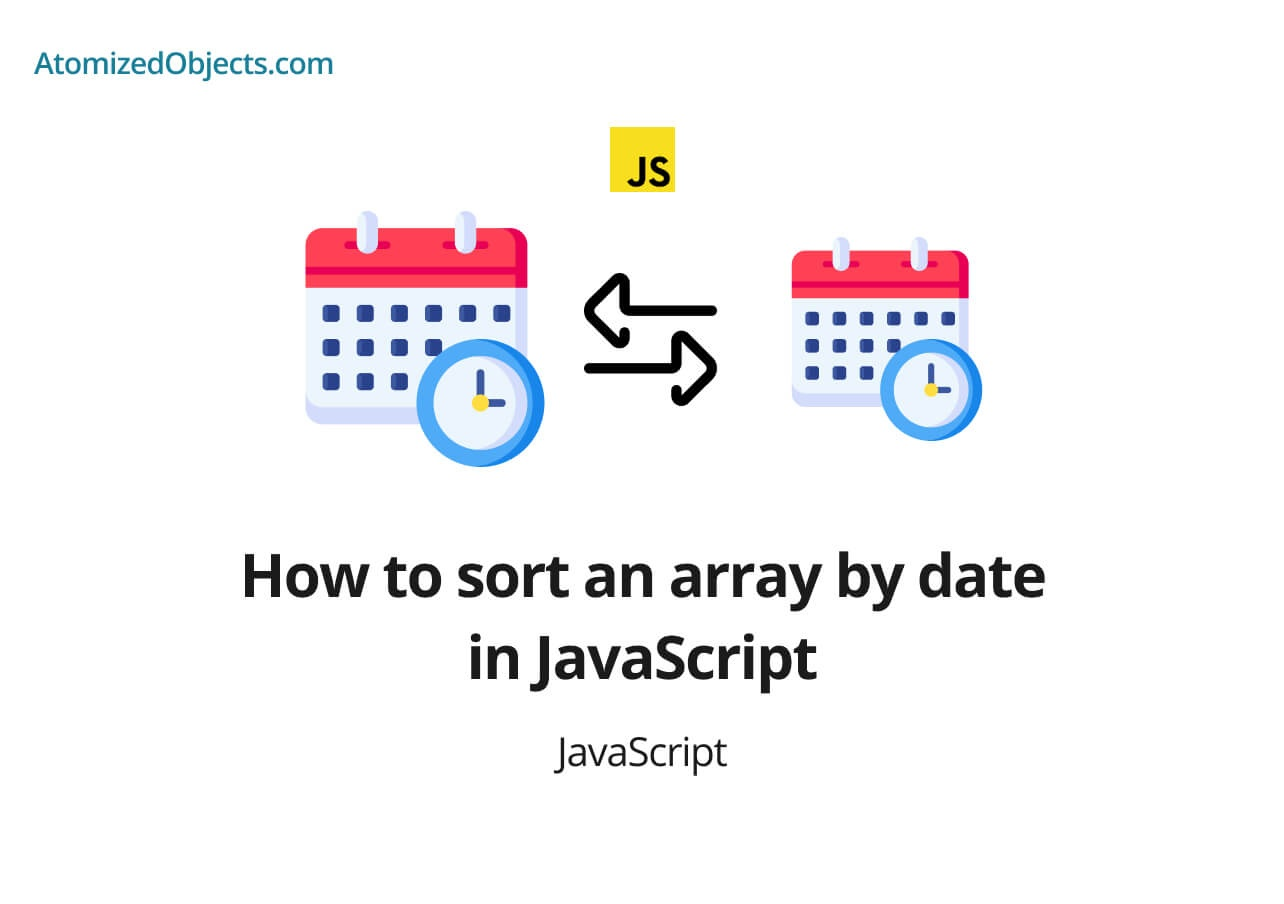Blog / Javascript

# How to sort an array by date in JavaScriptIn this post find out about how to sort an array by date in JavaScript as well as sorting in descending order, and by timestamps.Will Mayger
September 02, 2021
Article

In this post we will be covering how to sort an array by date in JavaScript with as little technical jargon as possible so you will have everything you need right here without having to look any further!

To sort an array by date in JavaScript is actually fairly easy and simple to do because JavaScript will handle most of the work for us.

This post will go over how to sort an array by date in JavaScript as well as how to sort these dates in descending order.

This will involve a small amount of sorting with an array of objects and comparing dates in JavaScript.

## How to sort an array by date in JavaScript

The easiest way to sort an array by date in JavaScript is to use the Array.prototype.sort method along with subtracting one JavaScript date from another.

Here is an example of how this method of sorting an array by date in JavaScript looks:

``````const arrayOfDates = [
{ date: new Date("12/25/2020") },
{ date: new Date("12/24/2020") },
{ date: new Date("12/26/2020") },
]

const sortedDates = arrayOfDates.sort((dateA, dateB) => dateA.date - dateB.date)
// [{date: "12/24/2020" }, { date: "12/25/2020" }, { date: "12/26/2020" }]``````

And here is another example of sorting an array by date but without the objects:

``````const arrayOfDates = [
new Date("12/25/2020"),
new Date("12/24/2020"),
new Date("12/26/2020"),
]
const sortedDates = arrayOfDates.sort((dateA, dateB) => dateA - dateB)
// ["12/24/2020", "12/25/2020", "12/26/2020"]``````

And, here is one last example of sorting an array by date in JavaScript but this time if you have an array of strings instead of dates:

``````const arrayOfDates = ["12/25/2020", "12/24/2020", "12/26/2020"]
const sortedDates = arrayOfDates.sort(
(dateA, dateB) => new Date(dateA) - new Date(dateB)
) // ["12/24/2020", "12/25/2020", "12/26/2020"]``````

The reason this works is because when you subtract one date from another in JavaScript it automatically gets converted into a timestamp which is a number that we can use for numerical comparisons.

The Array.prototype.sort method accepts a callback where you need to return a positive or negative number that it can then use to determine the order of the array.

So by subtracting date a from date b we will see which should come after the other if the outcome of that sum is a positive number, a negative number or 0 if the dates are exactly equal.

One thing worth noting is that when you use the Array.prototype.sort method it will return a new array, but it will also sort the original array as well, for most of us this will not be a problem, but if it is you just need to make sure you protect the original array before calling sort on it.

Here are a few examples of how you can prevent the sort method from sorting the original array:

``````const arrayOfDates = [
{ date: new Date("12/25/2020") },
{ date: new Date("12/24/2020") },
{ date: new Date("12/26/2020") },
]

// protect the original array with one of these:
const sortedArray1 = [...arrayOfDates].sort(
(dateA, dateB) => dateA.date - dateB.date
)

const sortedArray2 = arrayOfDates
.map(a => a)
.sort((dateA, dateB) => dateA.date - dateB.date)

const sortedArray3 = arrayOfDates
.slice()
.sort((dateA, dateB) => dateA.date - dateB.date)``````

### How to sort an array by date in descending order in JavaScript

To order an array of dates in descending order in JavaScript we just need to invert the subtraction we are doing.

So instead of subtracting date b from date a, instead we need to subtract date a from date b.

Here is an example of how to sort an array by date in descending order in JavaScript:

``````const arrayOfDates = [
{ date: new Date("12/25/2020") },
{ date: new Date("12/24/2020") },
{ date: new Date("12/26/2020") },
]

const sortedDates = arrayOfDates.sort((dateA, dateB) => dateB.date - dateA.date)
// [{date: "12/26/2020" }, { date: "12/25/2020" }, { date: "12/24/2020" }]``````

### Summary

There we have how to sort an array by date in JavaScript, if you want more like this be sure to check out some of my other posts!Foxi

Budget Planner & Tracker

More money in your pocket by the end of the month.

Free to use and no account needed.

Get started now.

Some graphics used on this post were made using icons from flaticon.